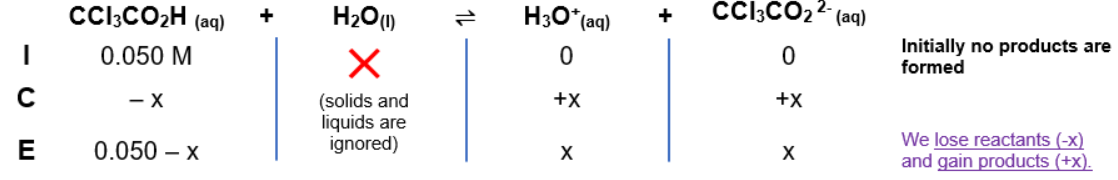# Problem: Trichloroacetic acid (CCl3CO2H) is a corrosive acid that is used to precipitate proteins. The pH of a 0.050-M solution of trichloroacetic acid is the same as the pH of a 0.040-M HClO4 solution. Calculate Ka for trichloroacetic acid.

###### FREE Expert Solution

HClO→ strong acid

pH = 1.398 (same pH as CCl3CO2H)

Remember that weak acids partially dissociate in water and that acids donate H+ to the base (water in this case).

The dissociation of CCl3CO2H is as follows:

CCl3CO2H(aq) + H2O(l)  H3O+(aq) + CCl3CO2(aq)

ICE Table:Ka expression:

$\overline{){{\mathbf{K}}}_{{\mathbf{a}}}{\mathbf{=}}\frac{\mathbf{products}}{\mathbf{reactants}}{\mathbf{=}}\frac{\mathbf{\left[}{\mathbf{H}}_{\mathbf{3}}{\mathbf{O}}^{\mathbf{+}}\mathbf{\right]}\mathbf{\left[}{\mathbf{CCl}}_{\mathbf{3}}{{\mathbf{CO}}_{\mathbf{2}}}^{\mathbf{-}}\mathbf{\right]}}{\mathbf{\left[}{\mathbf{CCl}}_{\mathbf{3}}{\mathbf{CO}}_{\mathbf{2}}\mathbf{H}\mathbf{\right]}}}$

Solving for [H3O+] from pH:

$\overline{){\left[}{{H}}_{{3}}{{O}}^{{+}}{\right]}{\mathbf{=}}{{\mathbf{10}}}^{\mathbf{-}\mathbf{pH}}}$

${\mathbf{\left[}}{{\mathbf{H}}}_{{\mathbf{3}}}{{\mathbf{O}}}^{{\mathbf{+}}}{\mathbf{\right]}}\mathbf{=}{\mathbf{10}}^{\mathbf{-}\mathbf{1}\mathbf{.}\mathbf{398}}$

[H3O+ = 0.040 M

89% (185 ratings)###### Problem Details

Trichloroacetic acid (CCl3CO2H) is a corrosive acid that is used to precipitate proteins. The pH of a 0.050-M solution of trichloroacetic acid is the same as the pH of a 0.040-M HClO4 solution. Calculate Ka for trichloroacetic acid.

Frequently Asked Questions

What scientific concept do you need to know in order to solve this problem?

Our tutors have indicated that to solve this problem you will need to apply the Weak Acids concept. If you need more Weak Acids practice, you can also practice Weak Acids practice problems.

What professor is this problem relevant for?

Based on our data, we think this problem is relevant for Professor Costanza's class at USF.

What textbook is this problem found in?

Our data indicates that this problem or a close variation was asked in Chemistry: An Atoms First Approach - Zumdahl Atoms 1st 2nd Edition. You can also practice Chemistry: An Atoms First Approach - Zumdahl Atoms 1st 2nd Edition practice problems.